Common Core: 7.SP.7a 7.SP.8a S.CP.2 S.CP.8

# "And" Probability for Independent Events

Author: Sophia Tutorial
##### Description:

Calculate an "AND" probability for independent events.

(more)

Sophia’s self-paced online courses are a great way to save time and money as you earn credits eligible for transfer to many different colleges and universities.*

No credit card required

37 Sophia partners guarantee credit transfer.

299 Institutions have accepted or given pre-approval for credit transfer.

* The American Council on Education's College Credit Recommendation Service (ACE Credit®) has evaluated and recommended college credit for 32 of Sophia’s online courses. Many different colleges and universities consider ACE CREDIT recommendations in determining the applicability to their course and degree programs.

Tutorial
what's covered
This tutorial will explain the rule for finding the probability of two or more independent events all happening. Our discussion breaks down as follows:

1. “And” Probability For Independent Events

## 1. “And” Probability

"And" means that both events A and B will occur, and they're going to be independent. There are different "and" probability formulas for independent events versus dependent events, but our tutorial today is going to focus on independent events.

EXAMPLE

Suppose a coin is flipped and this spinner is spun, where A and D are a lot more common than B or C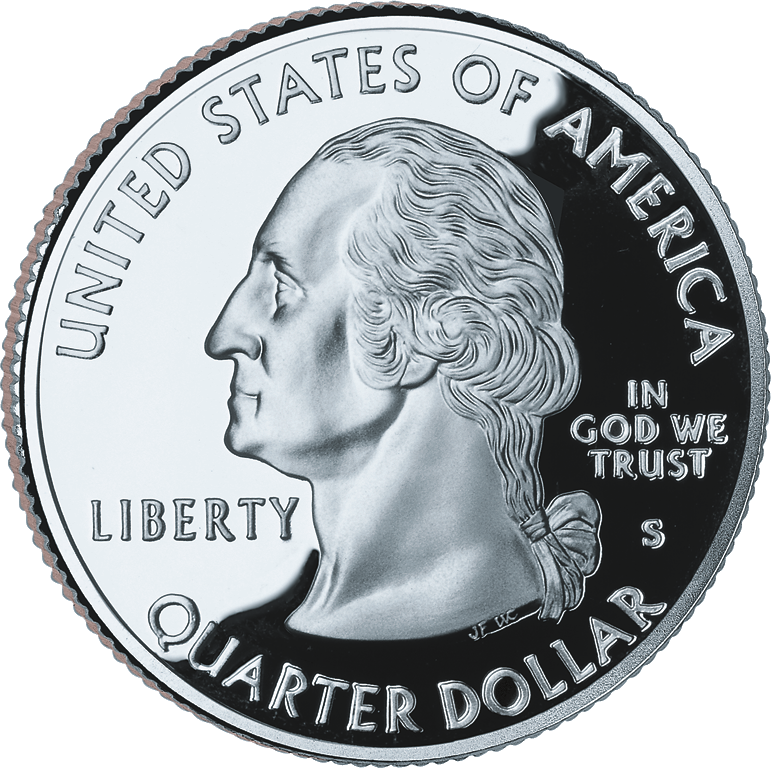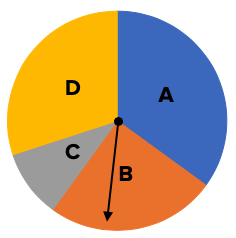Since the outcome of the coin flip doesn't have any influence on the probabilities for the spinner, these events are called independent. Spinning the spinner wouldn't have any effect on the probability of the coin coming up heads, and knowing if the coin came up heads doesn't affect the probabilities for the spinner.

You can create a tree diagram to show all the possible outcomes: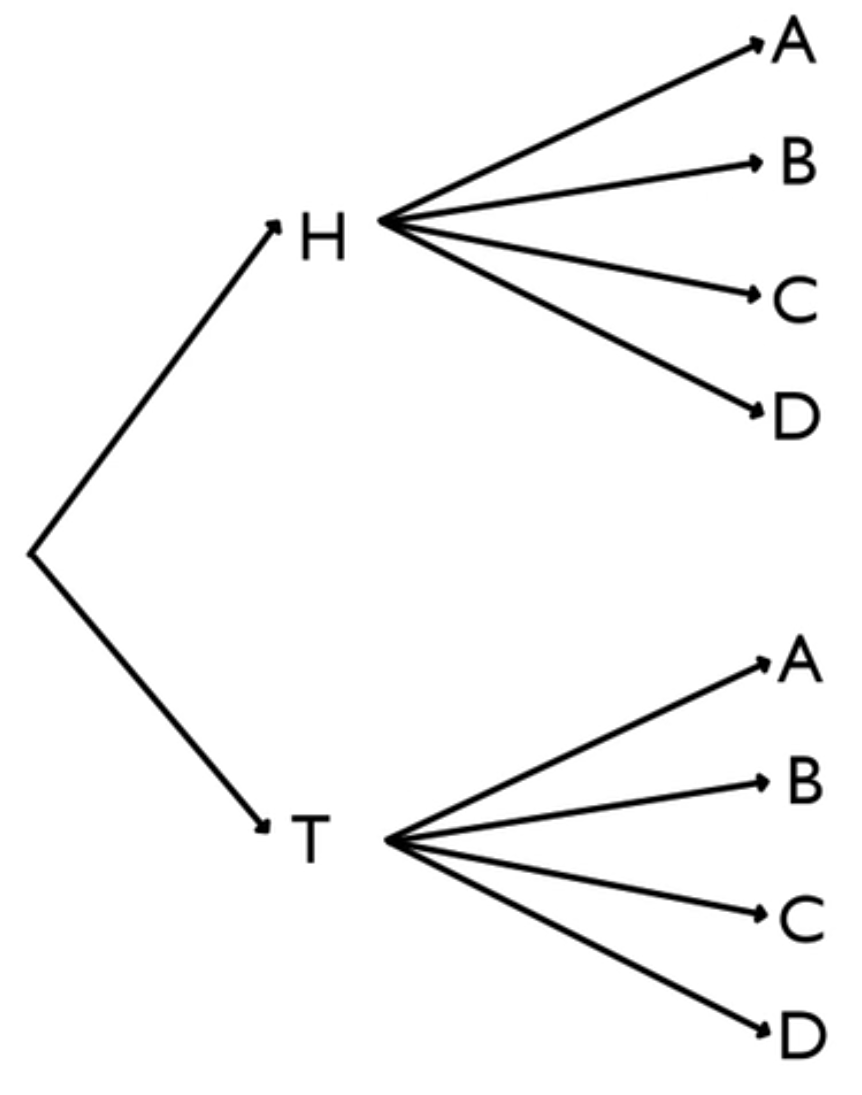There are two outcomes for the quarter, and there are four outcomes for the spinner. Using the Fundamental Counting Principle, we know that there are 2 times 4, or 8 total outcomes. However, they're not all equally likely.

The heads and tails each have a one-half probability, but A, B, C, and D don't all have the same probability. If you look closely at the spinner, A is the biggest sector with 35%, followed by D with 30%, then B with 25% and C with 10%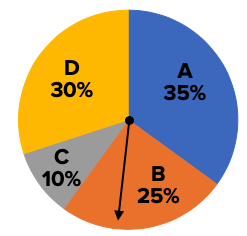Since it doesn't matter whether you got heads the first time or tails the first time--the probabilities will remain the same--you can apply the ratios down below too.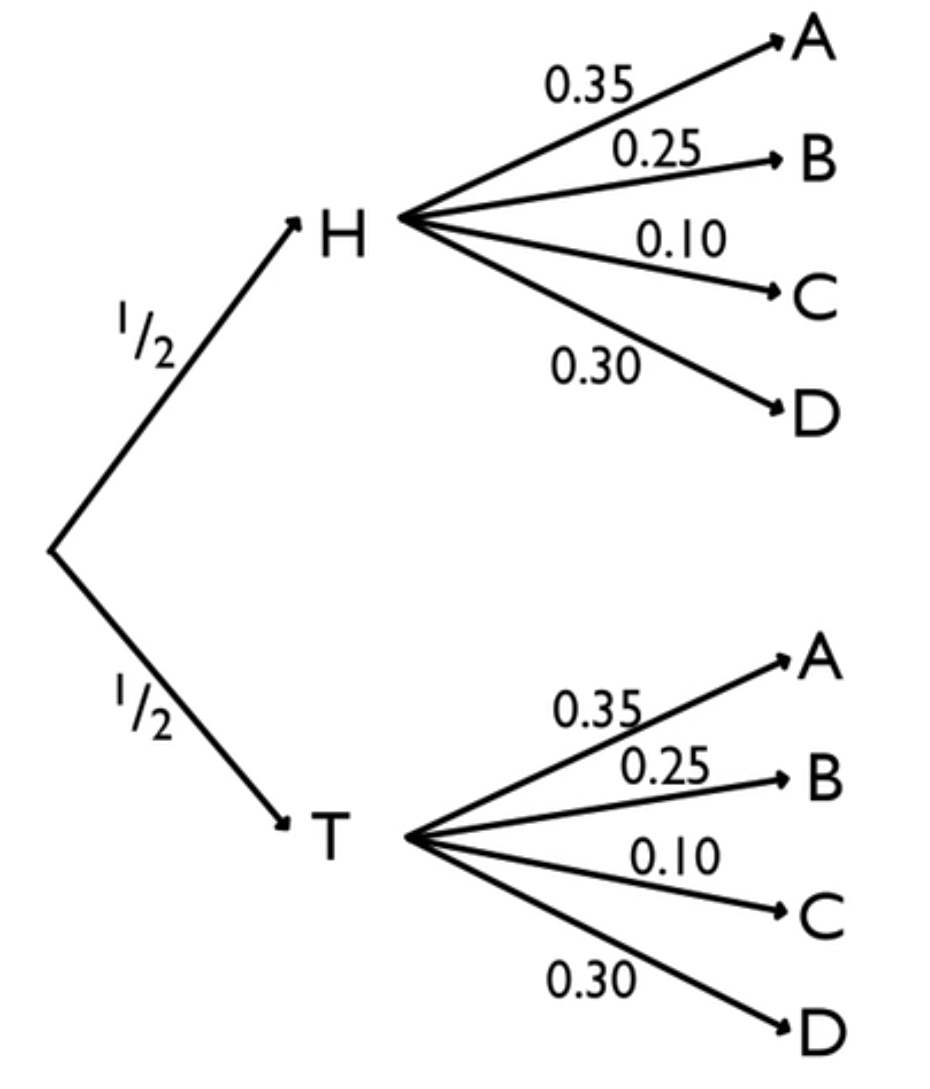Suppose that you did this experiment 1,000 times. You would expect about 500 heads and 500 tails if everything went according to your expectation.

If the second group went according to your expectation, 35% of those 500 would say A, 25% of those 500 would say B, 10% of those 500 would say C, and 30% of those 500 would say D.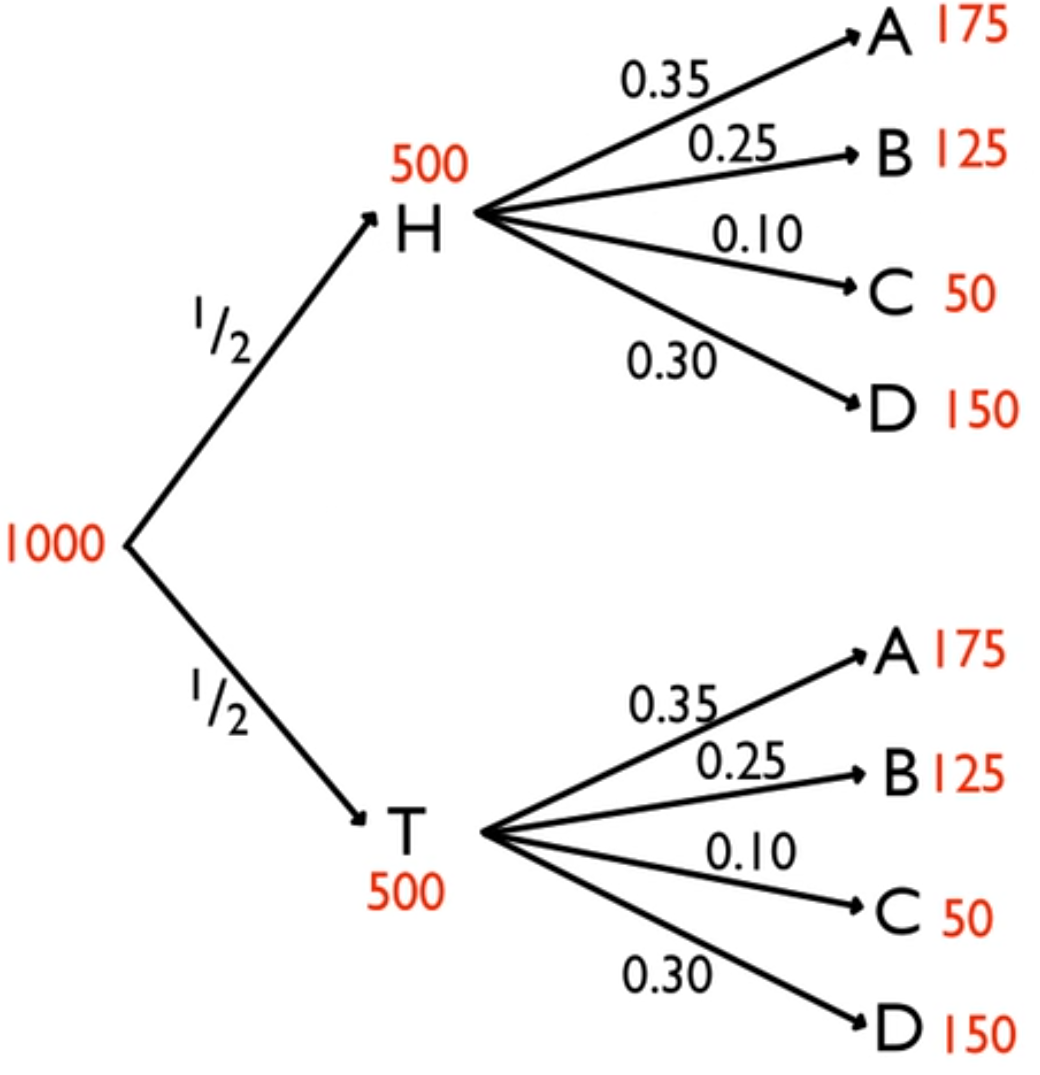Ultimately, you would end up with 175 of the 1,000 that went flipped heads and landed on A, 125 of the 1,000 that went flipped heads and landed on B, etc.

But what if you wanted to know the probability of flipping heads and landing on D? This would be 150 of the 1,000, and when simplified, equals 0.15.

Is there another way to obtain 0.15?

Look at the path that you traveled to get here. You went from heads down to D. You had those probabilities of 0.5 and 0.3, and the probability of H and D was 0.15. Hopefully, what you're seeing is that these two values multiplied equals 0.15.

In this case, it was 1/2 for heads and 0.3 for D. Multiply them, and you get 0.15.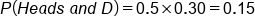This is a multiplication for independent events. The "and" probability for independent events, which is the probability of independent events A and B both happening, is the product of their individual probabilities.

formula
"And" Probability for Independent Events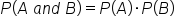term to know

"And" Probability for Independent Events
A way to approximate probability based on trials of chance experiments that mimic the real-life trials. Also known as the "Special Multiplication Rule".

summary
There is a special multiplication rule for finding "and" probability (also called joint probability) for two independent events. The probability that they both occur is equal to the product of their individual probabilities. Note, there is a different multiplication rule if the events aren't independent.

Good luck!

Source: Adapted from Sophia tutorial by Jonathan Osters.

Terms to Know
"And" Probability for Independent Events

A way to approximate probability based on trials of chance experiments that mimic the real-life trials.  Also known as the "Special Multiplication Rule".

Formulas to Know
"And" Probability for Independent EventsRating